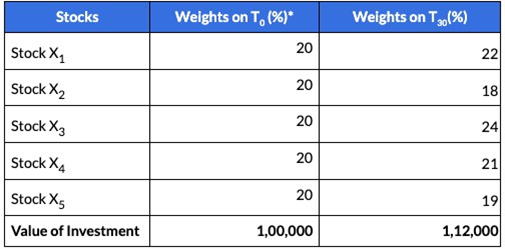# How does an SIP work in Equity Baskets?

Understanding the SIP Algorithm with an example:

The below table shows the composition on the day of investment and on the 1st SIP instalment. The change in weights is due to the change in stock prices over time.

Please note: To simplify, we have assumed an equal-weighted basket. The logic would work the same way for custom weights too

Initial Investment amount: 1,00,000

Monthly SIP instalment amount: 20,0001.      Calculate the number of shares based on the Total amount (Current value + SIP amount)
1. On the day SIP is due the current value of your investment is 1,12,000. We calculate the total amount as (Current Value + SIP amount) = 1,32,000
2. As per the above amount and the weights on T0, the number of shares to be bought will be calculated

2.      Calculate additional shares to be bought

1. We then calculate the difference from existing holdings to form your SIP instalment

3.      Determine the SIP amount

1. The stocks that have deviated below from the ideal composition are preferred
2. The actual instalment amount is calculated as New no. of shares * Market price of each stock

4.      In the next SIP instalment, the same steps are repeated

1. Every Equity Basket has an ideal weighting scheme
2. With time, as prices change, the constituents will deviate from the ideal composition. The algorithm compares your current constituents with the ideal composition to find the constituents that deviate the most
3. The SIP amount is first invested in stocks where the weightage might have reduced. With every instalment, the algorithm ensures your constituents try to match the ideal composition in the long run.